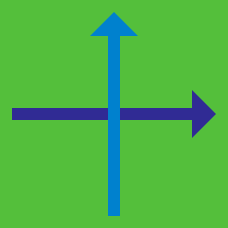Geometry

# Determining Coordinates

$ABC$ is a right triangle with $\angle ABC = 90 ^ \circ$.

If $A = ( 4, 9 )$ and $B = ( 11, 14)$, which of the following is a possible coordinate for $C$?

The point $P:(4, -26)$ is reflected across the origin, and now becomes the point $P':(a,b)$.

What is $a + b$?

3 out of 4 vertices of a square have coordinates $( 5, 6), (5, 9)$ and $(8, 6 )$. What is the coordinate of the last vertex?

$ABCD$ is a parallelogram. If $A = (0,0)$, $B = ( 4, 9 )$, and $C = ( 10, 14 )$, what are the coordinates of $D$?

$ABCD$ is a quadrilateral such that $AB \parallel CD$. $A = (0,0)$, $B = (2, 9)$, $C = ( 13, 20 )$, which of the following is a possible coordinate of $D$?

×